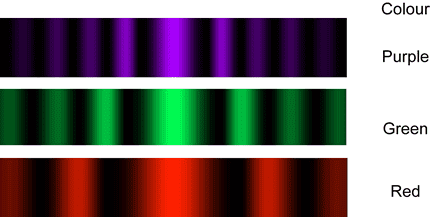# Diffraction Grating ratio

## Homework Statement

The same diffraction grating is used with two different wavelengths of light, λA and λB. The fourth-order principal maximum of light A exactly overlaps the third-order principal maximum of light B. Find the ratio λA/λB.

## Homework Equations

sin theta = m lambda / width of the slits

## The Attempt at a Solution

sin theta 1 = sin theta 2 since they overlap.

3/4

I don't understand how can 4th order principal and 3rd order principle can have the same sin theta? Because, unless I am misunderstanding something, I don't think it's possible for different orders to completely overlap on top of each other so that they meet at the same point on the opposite screen of the diffraction grating.

Simon Bridge
Homework Helper
The spacing between fringes depends on the wavelength.
This would happen if, say, light A created fringes 3deg apart and light B 4deg apart - the third fringe of B would be at 12deg which is the same as the 4th fringe of A.

In this case - you are correct:
$4\lambda_A = 3\lambda_B \Rightarrow \frac{\lambda_A}{\lambda_B}=\frac{3}{4}$

This result demonstrates the conditions required for this to happen.

eg. if λA=330nm then λB=440nm

Ideally I should show you:... the green 2nd order matches with the purple 3rd order. If we tweaked the wavelength only slightly it would be as exact as you like.

Last edited: